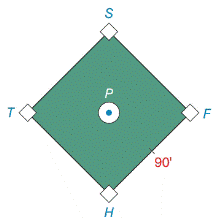Chapter 11.4, Problem 39EElementary Geometry For College St...

7th Edition
Alexander + 2 others
ISBN: 9781337614085

Solutions

Chapter
SectionElementary Geometry For College St...

7th Edition
Alexander + 2 others
ISBN: 9781337614085
Textbook Problem

On the baseball diamond, the pitcher’s mound is 60  ft , 6 in. from home plate. Bases are 90  ft apart. Use the Law of Cosines to find the distance from the pitcher’s mound to first base.Exercises 38 – 39

To determine

To find:

The distance from the pitcher’s mound to first base of the baseball diamond whose bases are 90 ft and pitcher’s mound is 60 ft,6in from the home plate.

Explanation

1) Formula:

The Law of Cosines

a2=b2+c22bccosα

b2=a2+c22accosβ

c2=a2+b22abcosγ

Where α, β, and γ are the acute angles of the triangle, and a, b, and c are the sides of the triangle.

2) Calculation:

Given,

From the figure

P is the pitcher mound and R is the home plate of the baseball diamond which is a square 90ft on a side.

The distance from pitcher mound to the home plate is RP¯=60ft.6in.

RP¯=60ft+12ft

[since, 6 in=12ft]

=(60+12)ft

=60+0.5ft

RP¯=60.5ft

Then, the figure is,

From the triangle RPF,

γ=P=90

Let a=PF¯, b=60

Still sussing out bartleby?

Check out a sample textbook solution.

See a sample solution

The Solution to Your Study Problems

Bartleby provides explanations to thousands of textbook problems written by our experts, many with advanced degrees!

Get Started